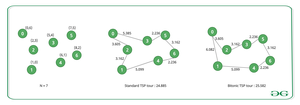Related Articles

# Bitonic Travelling Salesman Problem

• Last Updated : 13 Jul, 2021

Given a 2D array, arr[][] denoting a list of coordinates of N vertices on 2D space that is already sorted by x-coordinates and y-coordinates, the task is to find the minimum distance of a tour that starts from the leftmost vertex, and strictly goes to the right, and then upon reaching the rightmost vertex, the tour goes strictly from right to left-back to the starting vertex.

Examples:

Input: N = 7, arr[][] = {{0, 6}, {1 0}, {2 3}, {5 4}, {6 1}, {7 5}, {8 2}}
Output: 25.582
Explanation:The TSP tour: 0-3-5-6-4-1-2-0 is not a Bitonic TSP tour because although the tour initially goes from left to right (0-3-5-6) and then goes back from right to left (6-4-1), it then makes another left to right (1-2) and then right to left (2-0) steps.
The tour: 0-2-3-5-6-4-1-0 is a valid Bitonic TSP tour because it can be decomposed into two paths: 0-2-3-5-6 that goes from left to right and 6-4-1-0 that goes back from right to left.

Input: N = 3, arr[][] = {{1, 1}, {2, 3}, {3, 1}}
Output: 6.47

Approach: The above problem can be solved using Dynamic Programming. For the sake of understanding, the problem can be changed into two people. Both should start from the leftmost point at the same time. Walk along two different paths, and finally reach the rightmost point, except for the starting point and the endpoint.

• Every point happens to be passed by one person. Here, dp[i][j] represents how far the first person walks to i and the second person walks to j.
• In the solution, dp[i][j] means that 1 to max(i, j) have all been walked, and the current positions of the two people are i and j respectively, and how far they need to go.
• Also, it can be inferred that dp[i][j] is equal to dp[j][i], so from now on it is stipulated that i is always greater than j i.e. i>j in the state.
• In this way, no matter that person, the next step can only go to i+1, i+2,… these points.
• So, the state dp[i][j] can only be transferred to dp[i+1][j] or dp[i][i+1].

Follow the steps below to solve the problem:

• Create a 2D array, dp[][] of size N*N.
• Iterate the last row of the table, dp, and update dp[N-1][i] to the sum of distance(N-1, N) and distance(i, N), where distance(x, y) represents the Euclidean distance between xth and yth points of arr.
• Create a recursive function findTour(i, j) to fill all other cells
• Update dp[i][j] to minimum of findTour(i+1, j)+distance(i, i+1) and findTour(i+1, i)+distance(j, i+1).

Below is the implementation of the above approach:

## C++

 `// C++ program for the above approach``#include ``using` `namespace` `std;` `// Size of the array a[]``const` `int` `mxN = 1005;` `// Structure to store the x and``// y coordinates of a point``struct` `Coordinates {``    ``double` `x, y;``} a[mxN];` `// Declare a 2-D dp array``float` `dp[mxN][mxN];` `// Function to calculate the``// distance between two points``// in a Euclidian plane``float` `distance(``int` `i, ``int` `j)``{``    ``// Return the distance``    ``return` `sqrt``(``      ``(a[i].x - a[j].x) * (a[i].x - a[j].x)``    ``+ (a[i].y - a[j].y) * (a[i].y - a[j].y));``}` `// Utility recursive function to find``// the bitonic tour distance``float` `findTourDistance(``int` `i, ``int` `j)``{``    ``// Memoization``    ``if` `(dp[i][j] > 0)``        ``return` `dp[i][j];` `    ``// Update dp[i][j]``    ``dp[i][j] = min(``    ``findTourDistance(i + 1, j) + distance(i, i + 1),``    ``findTourDistance(i + 1, i) + distance(j, i + 1));` `    ``return` `dp[i][j];``}` `// Function to find the``// bitonic tour distance``void` `bitonicTSP(``int` `N)``{``    ``// Initialize the dp array``    ``memset``(dp, 0, ``sizeof``(dp));` `    ``// Base Case``    ``for` `(``int` `j = 1; j < N - 1; j++)``        ``dp[N - 1][j] = distance(N - 1, N)``              ``+ distance(j, N);` `    ``// Print the answer``    ``printf``(``"%.2f\n"``, findTourDistance(1, 1));``}` `// Driver Code``int` `main()``{``    ``// Given Input``    ``int` `N = 3;``    ``a.x = 1, a.y = 1;``    ``a.x = 2, a.y = 3;``    ``a.x = 3, a.y = 1;` `    ``// Function Call``    ``bitonicTSP(N);``}`

Output
`6.47`

Time Complexity: O(N2)
Auxiliary Space: O(N2)My Personal Notes arrow_drop_up